## Example Questions

### Example Question #201 : Geometry

What is the slope of a line parallel to the line: -15x + 5y = 30 ?

3

-15

1/3

30

3

Explanation:

First, put the equation in slope-intercept form: y = 3x + 6. From there we can see the slope of this line is 3 and since the slope of any line parallel to another line is the same, the slope will also be 3.

### Example Question #1 : How To Find The Slope Of Parallel Lines

What is the slope of any line parallel to –6x + 5y = 12?

5/6

12/5

6/5

6

12

6/5

Explanation:

This problem requires an understanding of the makeup of an equation of a line.  This problem gives an equation of a line in y = mx + b form, but we will need to algebraically manipulate the equation to determine its slope.  Once we have determined the slope of the line given we can determine the slope of any line parallel to it, becasue parallel lines have identical slopes.  By dividing both sides of the equation by 5, we are able to obtain an equation for this line that is in a more recognizable y = mx + b form. The equation of the line then becomes y = 6/5x + 12/5, we can see that the slope of this line is 6/5.

### Example Question #11 : Coordinate Geometry

What is the slope of a line that is parallel to the line 11x + 4y - 2 = 9 – 4x  ?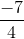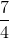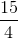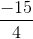Explanation:

We rearrange the line to express it in slope intercept form.

Any line parallel to this original line will have the same slope.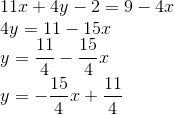### Example Question #1 : How To Find The Slope Of Parallel Lines

In the standard (x, y) coordinate plane, what is the slope of a line parallel to the line with equation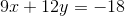?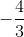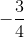Explanation:

Parallel lines will have equal slopes. To solve, we simply need to rearrange the given equation into slope-intercept form to find its slope.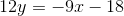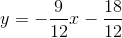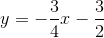The slope of the given line is. Any lines that run parallel to the given line will also have a slope of.

### Example Question #1 : How To Find The Slope Of Parallel Lines

What is the slope of a line that is parallel to the line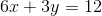?Explanation:

Parallel lines have the same slope. The question requires you to find the slope of the given function. The best way to do this is to put the equation in slope-intercept form (y = mx + b) by solving for y.

First subtract 6x on both sides to get 3y = –6x + 12.

Then divide each term by 3 to get y = –2x + 4.

In the form y = mx + b, m represents the slope. So the coefficient of the x term is the slope, and –2 is the correct answer.

### Example Question #1 : How To Find The Slope Of Parallel Lines

What is the slope of any line parallel to the line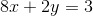?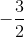Explanation:

To answer this question, we must find the slope of a line parallel to the line.

When a line is parallel to another, they have the same slope. Therefore, if we find the slope of the line we are given, we will find the slope of any line that would be parallel to it.

To find the slope, we must put our equation into point-intercept form. Point-intercept form is displayed as the following:, whereis the slope andis where the line intercepts the-axis.

Note that to put a line into point-intercept form, you must solve for.

Therefore, we must solve for. So, for this data, we must first subtract both sides of the equation by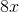: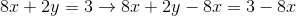This becomes: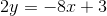Now we must divide each side byto getby itself: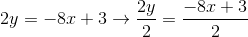This becomes: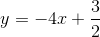Becauseisin our point-intercept form, the slope of our line is. Therefore, the slope of any line parallel to this line is also.

### Example Question #1 : How To Find The Slope Of Parallel Lines

What is the slope of a line parallel to the line given by the equation: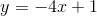?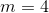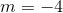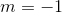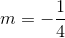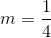Explanation:

Parallel lines have the same slope. You find slope by using the general form of slope-intercept:whererepresents the slope of the line andrepresents the-intercept.

For our equation we see that theisthus the anser is.

### Example Question #1 : How To Find The Slope Of Parallel Lines

What is the slope of a line parallel to the line defined by the equation: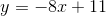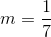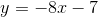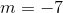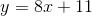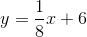Explanation:

The slope of a line in slope-intercept form is given by the coefficient,in the equation:. For two lines to be parallel, they have to have the same slope. Thus we see in our equation that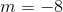and so a line that is parallel must also have a slope of### Example Question #1 : How To Find The Slope Of Parallel Lines

Which of the following is the equation of a line parallel to the line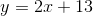.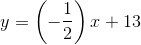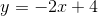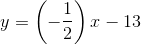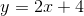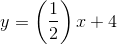Parallel lines have equivalent slopes, so the correct answer is.